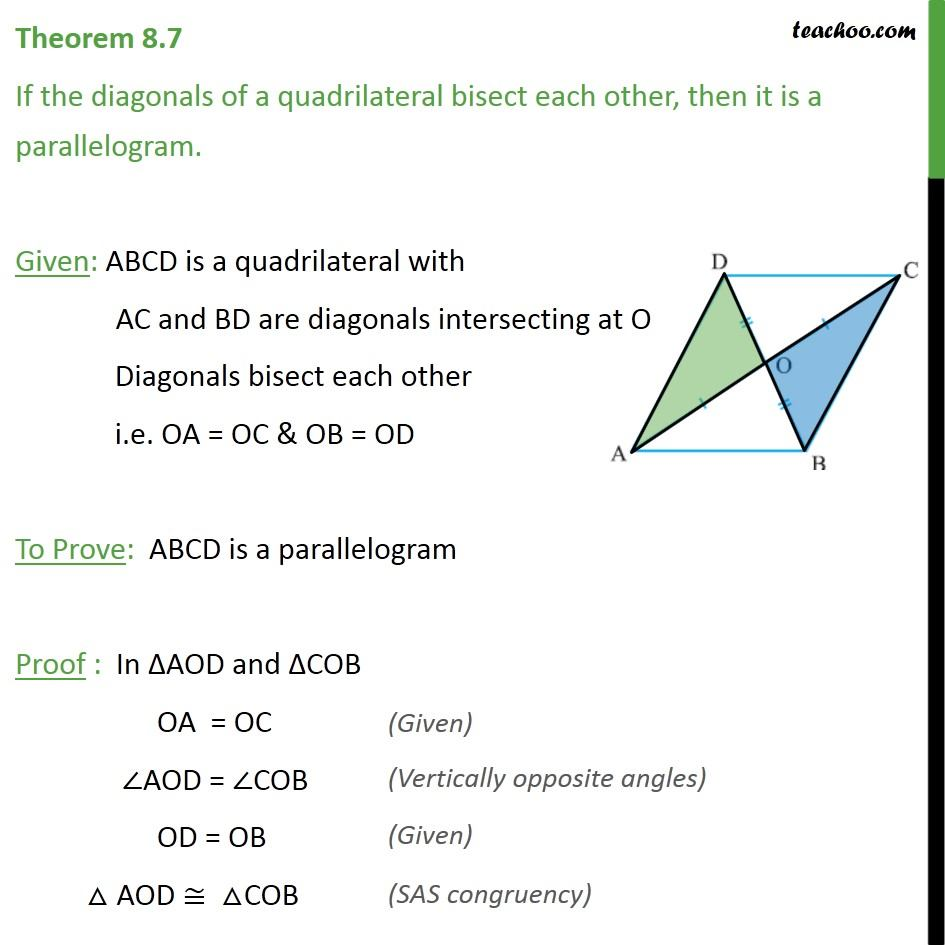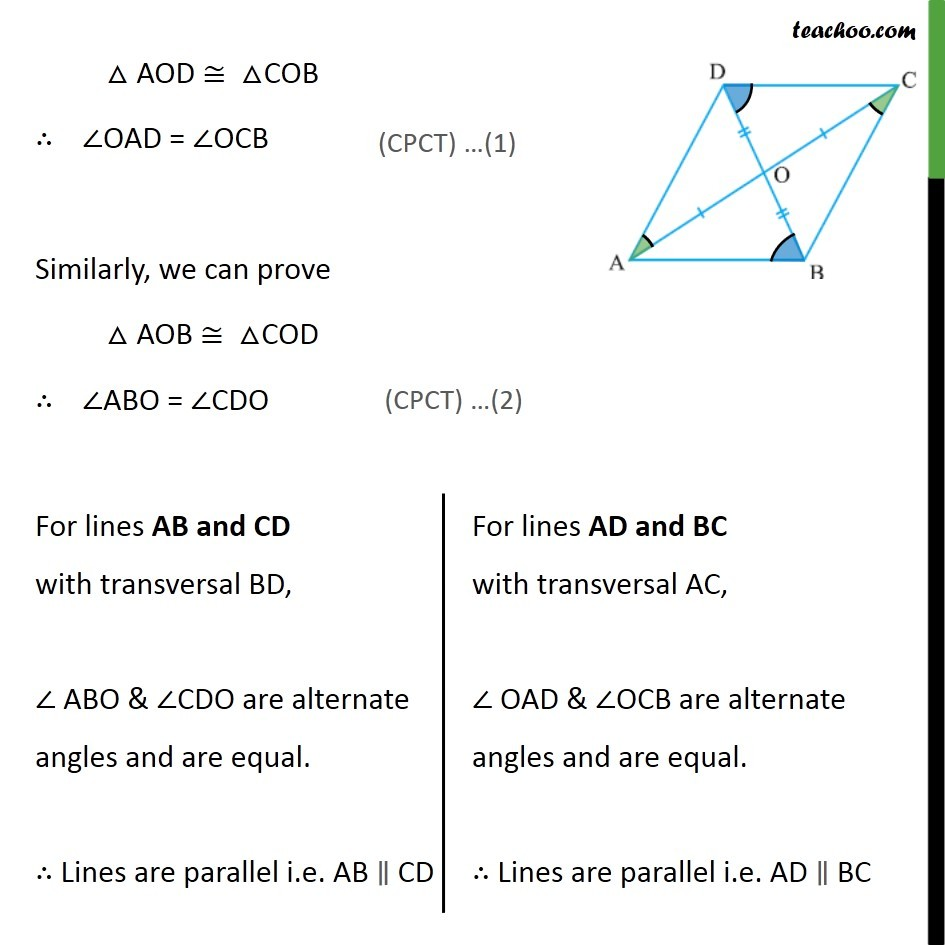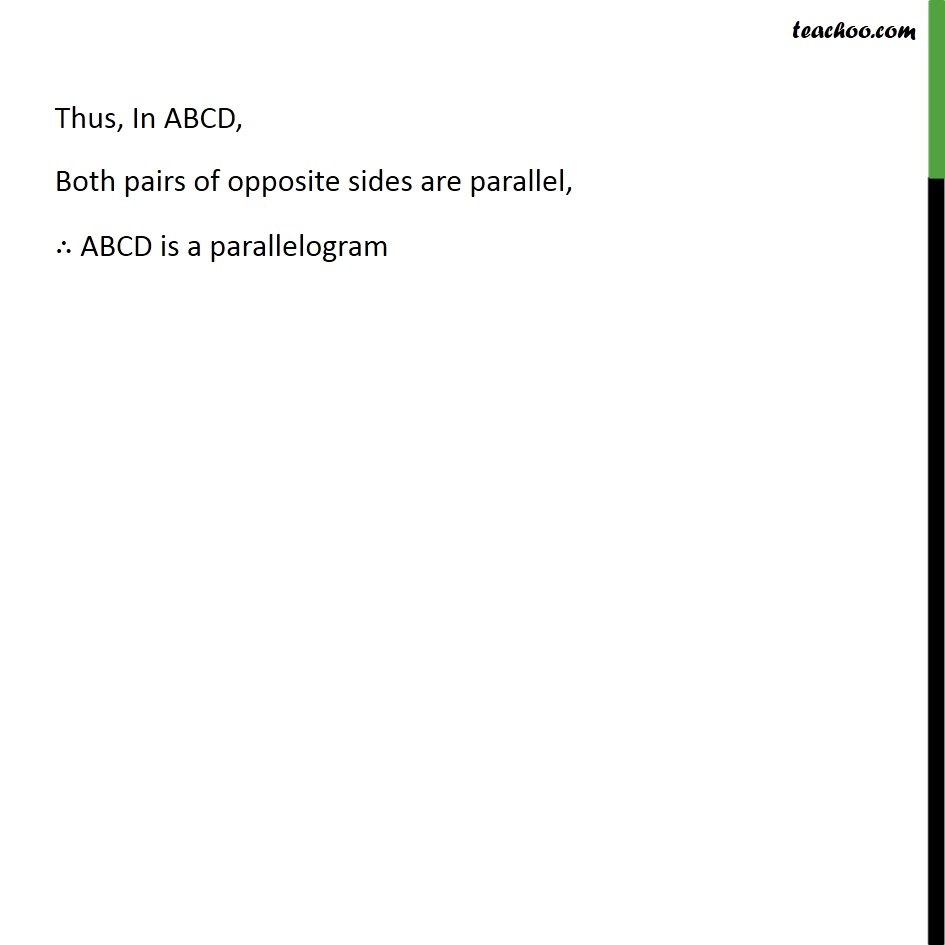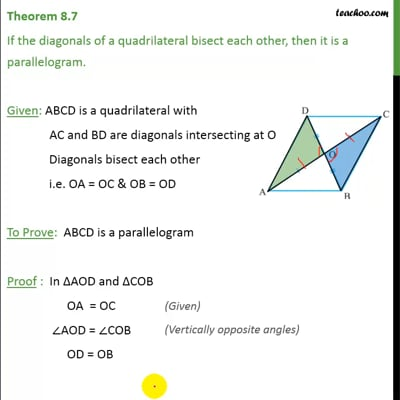Theorems

Chapter 8 Class 9 Quadrilaterals
Serial order wiseThis video is only available for Teachoo black users

Solve all your doubts with Teachoo Black (new monthly pack available now!)

### Transcript

Theorem 8.7 If the diagonals of a quadrilateral bisect each other, then it is a parallelogram. Given: ABCD is a quadrilateral with AC and BD are diagonals intersecting at O Diagonals bisect each other i.e. OA = OC & OB = OD To Prove: ABCD is a parallelogram Proof : In AOD and COB OA = OC AOD = COB OD = OB AOD COB ODA OBC OAD = OCB Similarly, we can prove AOB COD ABO = CDO Thus, In ABCD, Both pairs of opposite sides are parallel, ABCD is a parallelogram